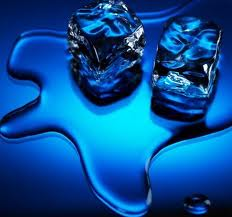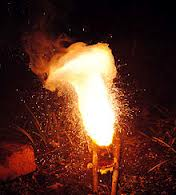Difference between Exothermic and Endothermic

Key Difference: In Chemistry, Exothermic and Endothermic are the two prominent types of reactions. An endothermic reaction requires heat (energy). On the other hand, an exothermic reaction is the one which releases heat (energy). Thus, both the reactions are just opposite to each other.

Whenever molecules interact with each other various reactions take place. These reactions are categorized into many groups according to their features or characteristics. Two of these reactions are known as exothermic and endothermic reactions.An endothermic reaction requires heat. On the other hand, an exothermic reaction is the one which releases heat. Thus, both the reactions are just opposite to each other. To find out in detail about these reactions one must learn the basic principles behind them. An endothermic reaction is that type of reaction which requires or needs energy. This energy is supplied from the surroundings. The amount of energy supplied to these reactions may vary.

One of the most common examples of these reactions is a photosynthesis reaction in which plants take energy from sunlight and then convert carbon dioxide and water into oxygen and sugar. The energy content of the reactants in this case is less than the products.An exothermic reaction is just the opposite of an endothermic reaction as it produces heat. In some of the exothermic processes, initially some amount of heat may be required to start up the process. Once supplied, later the system starts producing heat on its own. All the combustion reactions fall into the category of this type of reaction.

The two reactions can be simply understood by equations. Assume that after a reaction, A molecule generates B and C molecule. The energy of A remains greater than the total energy of B and C. In this case, the excessive energy will be released into the environment, and the reaction will be termed as an exothermic reaction.

A     --->  B + C + energy

(reactants)         (products)

Similarly, an endothermic reaction can be represented too. Lets assume that A needs to take some energy from its surroundings to form the product D. When the A molecule takes energy from the surrounding, then that particular surrounding becomes colder after the reaction. Melting of ice is an example of an endothermic reaction.

A + energy  ---> D

(reactants)        (product)

Comparison between Exothermic and Endothermic:

 Exothermic Endothermic Definition An exothermic reaction is just the opposite of an endothermic reaction as it produces heat. An endothermic reaction is that type of reaction which require or needs energy. This energy is supplied from the surroundings. Symbolic Equation A -->   B + C + energy A + energy  --> D Examples Digestion of food releases energy All combustion reactions (fires) Adding an alkali metal to water 2 Na + 2 H2O --> 2 NaOH + H2 + energy Melting of ice absorbs energy Dissolving ammonium nitrate in water (the essence of commercial cold packs) Energy Content Energy content of the products is more than than the reactants Energy content of the reactants is less than the products

Image Courtesy: wikipedia.org, blogs.cas.suffolk.edu